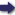(London :  Kegan Paul, Trench, Trübner & Co.,  1910.)

 Tools

## Search this bookPrev Page 24 Next``` ALBERUNTS INDIA. How many solar, lunar, and civil days are re¬ quired for the forma¬ tion of an adhimdsa month. The compu¬ tation of adhimdsa according to Pulisa. Page 215. lunar months is represented by these supernumerary months, by which a single year is extended to thirteen months. These, therefore, are the universal ccdhimdsa months. . The universal solar months of a kcdpa are 51,840, 000,000 ; the universal lunar months of a kalpta are 53,433,300,000. The difference between them or the adhimdsa, months is 1,593,300,000. Multiplying each of these numbers by 30, we get days, viz. solar days of a kalpa, 1,555,200,000,000; lunar days, i ,602,999,000,000 ; the days of the adhimdsa months, 47,799,000,000. In order to reduce these numbers to smaller ones we divide them by a common divisor, viz. 9,000,000. Thus we get as the sum of the days of the solar months 172,800 ; as the sum of the days of the lunar months, 178,111 ; and as the sum of the days of the adhimdsa months, 53ii. If we further divide the universal solar, civil, and lunar days of a kalpa, each kind of them separately, by the universal adhimdsa months, the quotient represents the number of days within which a whole adhimdsa month sums up, viz. in 976^%\*y solar days, in 1006J^^r^--^-^ lunar days, and in 990y|^||| civil days. This whole computation rests on the measures which Brahmagupta adopts regarding a kalp)a and the star- cycles in a kalpa. According to the theory of Pulisa regarding the caturyuga, a caturyuga has 51,840,000 solar months, 53,433,336 lunar months, 1,593,336 adhimdsa months. Accordingly a caturyuga has 1,555,200,000 solar days, 1,603,000,080 lunar days, 47,800,080 days of adhimdsa months. If we reduce the numbers of the months by the common divisor of 24, we get 2,160,000 solar months, 2,226,389 lunar months, 66,389 adhimdsa months. If we divide the numbers of the day by the common ```Prev Page 24 Next